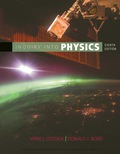# (Indicates a review question, which means it requires only a basic understanding of the material to answer. Questions without this designation typically require integrating or extending the concepts presented thus far.) In otherwise empty space, what would happen if the size of the electrostatic force acting between two positively charged objects was exactly the same as that of the gravitational force acting between them? What would happen if they were moved closer together or farther apart?### Inquiry into Physics

8th Edition
Ostdiek
Publisher: Cengage
ISBN: 9781337515863

#### Solutions

Chapter
Section### Inquiry into Physics

8th Edition
Ostdiek
Publisher: Cengage
ISBN: 9781337515863
Chapter 7, Problem 7Q
Textbook Problem
1 views

## (Indicates a review question, which means it requires only a basic understanding of the material to answer. Questions without this designation typically require integrating or extending the concepts presented thus far.) In otherwise empty space, what would happen if the size of the electrostatic force acting between two positively charged objects was exactly the same as that of the gravitational force acting between them? What would happen if they were moved closer together or farther apart?

To determine

If the size of the electrostatic force acting between two positively charged objects was exactly the same as the gravitational force acting between them, then what would happen. If they were moved closer together or farther apart?

### Explanation of Solution

The Universal Gravitation Equation states the force of attraction between two objects, where the mass is considered concentrated at their centers of mass:

F=GMm/R2         (1)

Where,

• F is the force of attraction between two objects.
• G is the Universal Gravitational Constant.
• M and  m are the masses of the two point objects.
• R is the separation between the centers of the objects.

The electrostatic force equation is called  Coulomb's Law  and states the force of attraction between particles of opposite electrical charge

### Still sussing out bartleby?

Check out a sample textbook solution.

See a sample solution

#### The Solution to Your Study Problems

Bartleby provides explanations to thousands of textbook problems written by our experts, many with advanced degrees!

Get Started

Find more solutions based on key concepts
Many sports drinks offer monosaccharides. a. that may help maintain hydration and contribute to blood glucose. ...

Nutrition: Concepts and Controversies - Standalone book (MindTap Course List)

Why don’t red dwarfs become giant stars?

Horizons: Exploring the Universe (MindTap Course List)

How might Vmax for an enzyme be determined?

Chemistry for Today: General, Organic, and Biochemistry

Provide two reasons why meiosis leads to genetic variation in diploid organisms.

Human Heredity: Principles and Issues (MindTap Course List)

A potential energy function for a system in which a two-dimensional force acts is of the form U = 3x3y 7x. Fin...

Physics for Scientists and Engineers, Technology Update (No access codes included)